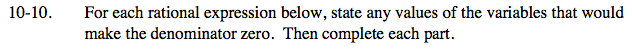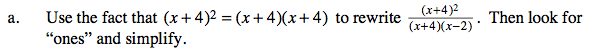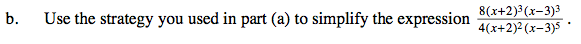### Home > AC > Chapter 10 > Lesson 10.1.1 > Problem10-10

10-10.For the denominator to equal zero (x + 4)(x − 2) must equal zero.

$\frac{(x+4)}{(x-2)}$What values of x would make the denominator zero?

Rewrite the expression, as shown below, and look for factors which simplify to one.

$\frac{8(x+2)(x+2)(x+2)(x-3)(x-3)(x-3)}{4(x+2)(x+2)(x-3)(x-3)(x-3)(x-3)(x-3)}$